This site is from a past semester! The current version will be here when the new semester starts.

# Modeling

This is a printer-friendly version. It omits exercises, optional topics (i.e., four-star topics), and other extra content such as learning outcomes.

## Introduction

#### What

A model is a representation of something else.

A class diagram is a model that represents a software design.

A model provides a simpler view of a complex entity because a model captures only a selected aspect. This omission of some aspects implies models are abstractions.

A class diagram captures the structure of the software design but not the behavior.

Multiple models of the same entity may be needed to capture it fully.

In addition to a class diagram (or even multiple class diagrams), a number of other diagrams may be needed to capture various interesting aspects of the software.

#### How

In software development, models are useful in several ways:

a) To analyze a complex entity related to software development.

Some examples of using models for analysis:

1. Models of the can be built to aid the understanding of the problem to be solved.
2. When planning a software solution, models can be created to figure out how the solution is to be built. An architecture diagram is such a model.

b) To communicate information among stakeholders. Models can be used as a visual aid in discussions and documentation.

Some examples of using models to communicate:

1. You can use an architecture diagram to explain the high-level design of the software to developers.
2. A business analyst can use a use case diagram to explain to the customer the functionality of the system.
3. A class diagram can be reverse-engineered from code so as to help explain the design of a component to a new developer.

c) As a blueprint for creating software. Models can be used as instructions for building software.

Some examples of using models as blueprints:

1. A senior developer draws a class diagram to propose a design for an OOP software and passes it to a junior programmer to implement.
2. A software tool allows users to draw UML models using its interface and the tool automatically generates the code based on the model.
Model Driven Development extra

#### UML Models

The following diagram uses the class diagram notation to show the different types of UML diagrams.source:https://en.wikipedia.org/

## Modeling structures

#### OO Structures

An OO solution is basically a network of objects interacting with each other. Therefore, it is useful to be able to model how the relevant objects are 'networked' together inside a software i.e. how the objects are connected together.

Given below is an illustration of some objects and how they are connected together. Note: the diagram uses an ad-hoc notation.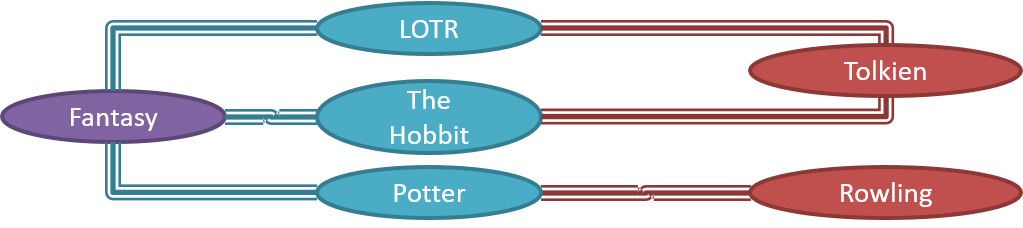Note that these object structures within the same software can change over time.

Given below is how the object structure in the previous example could have looked like at a different time.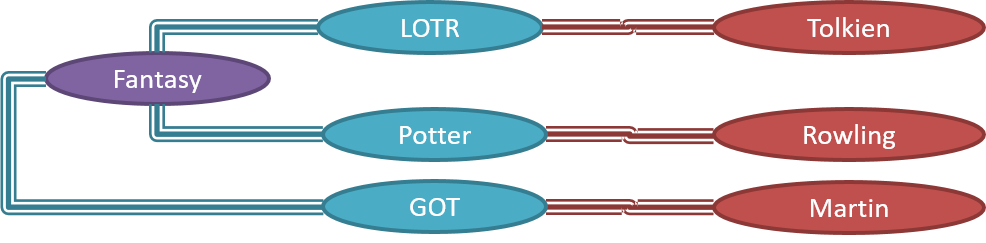However, object structures do not change at random; they change based on a set of rules, as was decided by the designer of that software. Those rules that object structures need to follow can be illustrated as a class structure i.e. a structure that exists among the relevant classes.

Here is a class structure (drawn using an ad-hoc notation) that matches the object structures given in the previous two examples. For example, note how this class structure does not allow any connection between `Genre` objects and `Author` objects, a rule followed by the two object structures above.UML Object Diagrams are used to model object structures and UML Class Diagrams are used to model class structures of an OO solution.

Here is an object diagram for the above example:And here is the class diagram for it: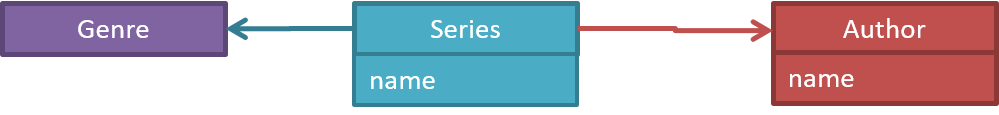#### Class Diagrams (Basics)

Contents of the panels given below belong to a different chapter; they have been embedded here for convenience and are collapsed by default to avoid content duplication in the printed version.

UML Class Diagrams → Introduction → What

Classes form the basis of class diagrams.

UML Class Diagrams → Classes → What

UML Class Diagrams → Class-Level Members → What

Associations are the main connections among the classes in a class diagram.

OOP Associations → What

UML Class Diagrams → Associations → What

The most basic class diagram is a bunch of classes with some solid lines among them to represent associations, such as this one.

An example class diagram showing associations between classes.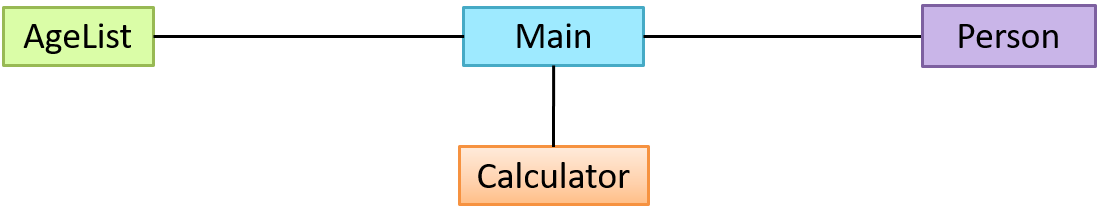UML Class Diagrams → Associations → Labels

UML Class Diagrams → Associations → Roles

OOP Associations → Multiplicity

UML Class Diagrams → Associations → Multiplicity

OOP Associations → Navigability

UML Class Diagrams → Associations → Navigability

Here is the same class diagram shown earlier but with some additional information included: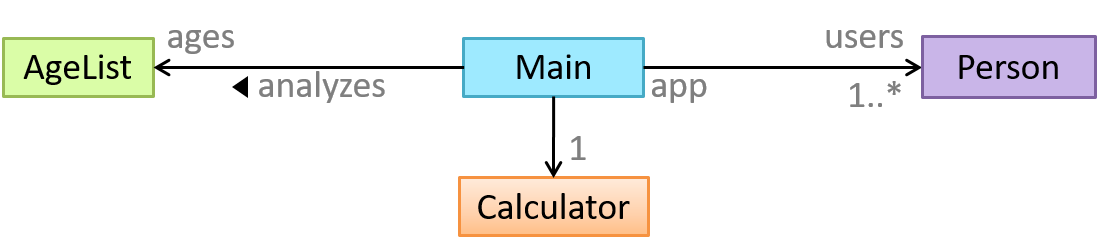UML → Notes

#### Class Diagrams - Intermediate

A class diagram can also show different types of relationships between classes: inheritance, compositions, aggregations, dependencies.

##### Modeling inheritance

OOP → Inheritance → What

UML → Class Diagrams → Inheritance → What

##### Modeling composition

OOP → Associations → Composition

UML → Class Diagrams → Composition → What

##### Modeling aggregation

OOP → Associations → Aggregation

UML → Class Diagrams → Aggregation → What

##### Modeling dependencies

OOP → Associations → Dependencies

UML → Class Diagrams → Dependencies → What

A class diagram can also show different types of class-like entities:

##### Modeling enumerations

OOP → Classes → Enumerations

UML → Class Diagrams → Enumerations → What

##### Modeling abstract classes

OOP → Inheritance → Abstract Classes

UML → Class Diagrams → Abstract Classes → What

##### Modeling interfaces

OOP → Inheritance → Interfaces

UML → Class Diagrams → Interfaces → What

A class diagram can show association classes too.

OOP → Associations → Association Classes

UML → Class Diagrams → Association Classes → What

#### Object Diagrams

UML → Object Diagrams → Introduction

Object diagrams can be used to complement class diagrams. For example, you can use object diagrams to model different object structures that can result from a design represented by a given class diagram.

UML → Object Diagrams → Objects

UML → Object Diagrams → Associations

#### Object Oriented Domain Models

The analysis process for identifying objects and object classes is recognized as one of the most difficult areas of object-oriented development. --Ian Sommerville, in the book Software Engineering

Class diagrams can also be used to model objects in the (i.e. to model how objects actually interact in the real world, before emulating them in the solution). Class diagrams that are used to model the problem domain are called conceptual class diagrams or OO domain models (OODMs).

The OO domain model of a snakes and ladders game is given below.

Description: The snakes and ladders game is played by two or more players using a board and a die. The board has 100 squares marked 1 to 100. Each player owns one piece. Players take turns to throw the die and advance their piece by the number of squares they earned from the die throw. The board has a number of snakes. If a player’s piece lands on a square with a snake head, the piece is automatically moved to the square containing the snake’s tail. Similarly, a piece can automatically move from a ladder foot to the ladder top. The player whose piece is the first to reach the 100th square wins.OODMs do not contain solution-specific classes (i.e. classes that are used in the solution domain but do not exist in the problem domain). For example, a class called DatabaseConnection could appear in a class diagram but not usually in an OO domain model because DatabaseConnection is something related to a software solution but not an entity in the problem domain.

OODMs represents the class structure of the problem domain and not their behavior, just like class diagrams. To show behavior, use other diagrams such as sequence diagrams.

OODM notation is similar to class diagram notation but omit methods and navigability.

## Modeling behaviors

#### Activity Diagrams - Basic

Software projects often involve workflows. Workflows define the flow in which a process or a set of tasks is executed. Understanding such workflows is important for the success of the software project.

Some examples in which a certain workflow is relevant to software project:

A software that automates the work of an insurance company needs to take into account the workflow of processing an insurance claim.

The algorithm of a piece of code represents the workflow (i.e. the execution flow) of the code.

UML Activity Diagrams → Introduction → What

UML Activity Diagrams → Basic Notation → Linear Paths

UML Activity Diagrams → Basic Notation → Alternate Paths

UML Activity Diagrams → Basic Notation → Parallel Paths

#### Activity Diagrams - Intermediate

UML Activity Diagrams → Intermediate Notation → Rakes

UML Activity Diagrams → Intermediate Notation → Swim Lanes

#### Sequence Diagrams - Basic

Sequence diagrams model the interactions between various entities in a system, in a specific scenario. Modelling such scenarios is useful, for example, to verify the design of the internal interactions is able to provide the expected outcomes.

Some examples where a sequence diagram can be used:

To model how components of a system interact with each other to respond to a user action.

To model how objects inside a component interact with each other to respond to a method call it received from another component.

UML Sequence Diagrams → Introduction

UML Sequence Diagrams → Basic Notation

UML Sequence Diagrams → Loops

UML Sequence Diagrams → Object Creation

UML Sequence Diagrams → Minimal Notation

#### Sequence Diagrams - Intermediate

UML Sequence Diagrams → Object Deletion

UML Sequence Diagrams → Self-Invocation

UML Sequence Diagrams → Alternative Paths

UML Sequence Diagrams → Optional Paths

UML Sequence Diagrams → Calls to Static Methods

#### Use Case Diagrams

Use case diagrams model the mapping between features of a system and its user roles i.e., which user roles can perform which tasks using the software.

A simple use case diagram: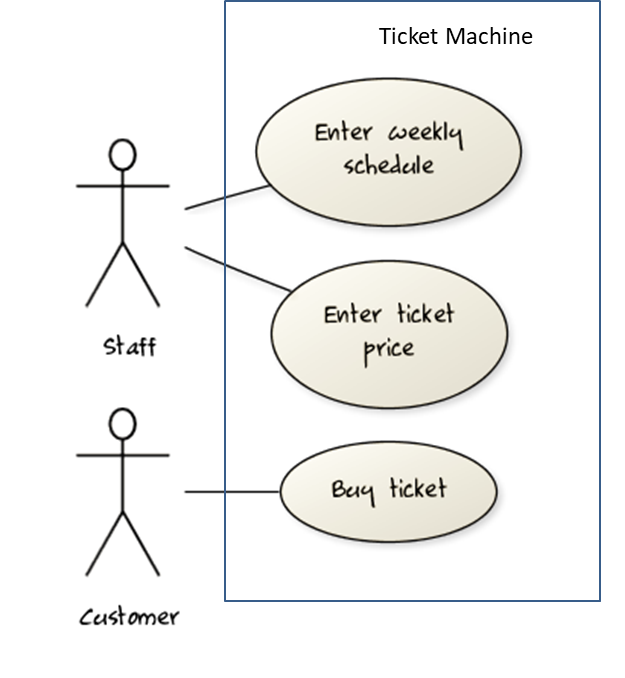## Modeling a solution

#### Introduction

You can use models to analyze and design software before you start coding.

Suppose you are planning to implement a simple minesweeper game that has a text based UI and a GUI. Given below is a possible OOP design for the game.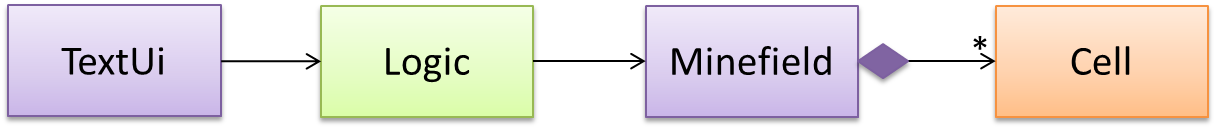Before jumping into coding, you may want to find out things such as,

• Is this class structure able to produce the behavior you want?
• What API should each class have?
• Do you need more classes?

To answer these questions, you can analyze how the objects of these classes will interact with each other to produce the behavior you want.

#### Basic

As mentioned in [Design → Modeling → Modeling a Solution → Introduction], this is the Minesweeper design you have come up with so far. Our objective is to analyze, evaluate, and refine that design.Let us start by modeling a sample interaction between the person playing the game and the `TextUi` object.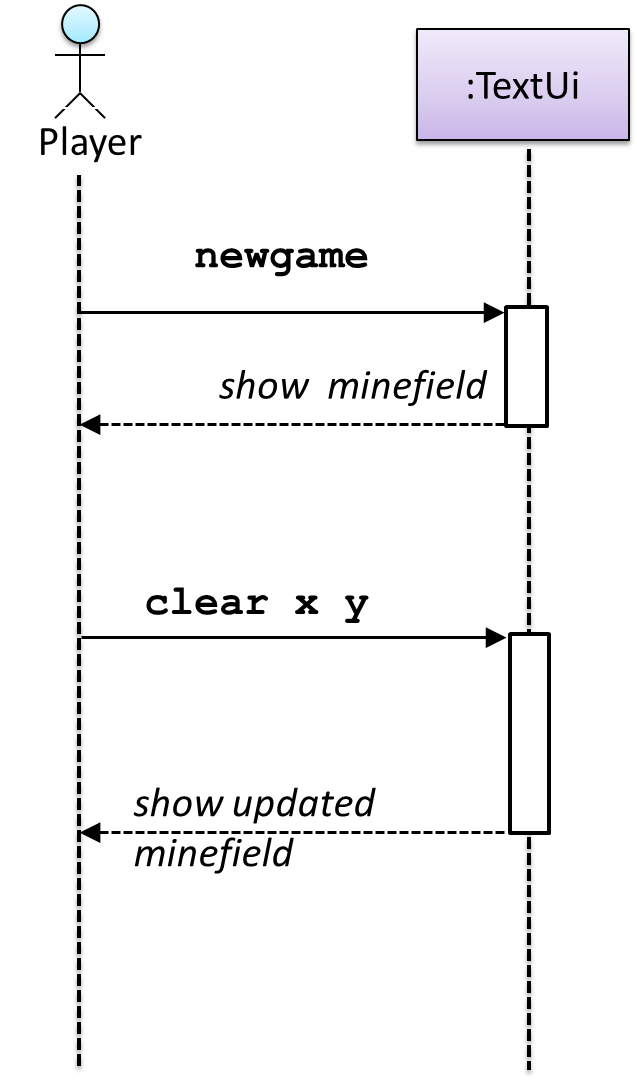`newgame` and `clear x y` represent commands typed by the `Player` on the `TextUi`.

How does the `TextUi` object carry out the requests it has received from the player? It would need to interact with other objects of the system. Because the `Logic` class is the one that controls the game logic, the `TextUi` needs to collaborate with `Logic` to fulfill the `newgame` request. Let us extend the model to capture that interaction.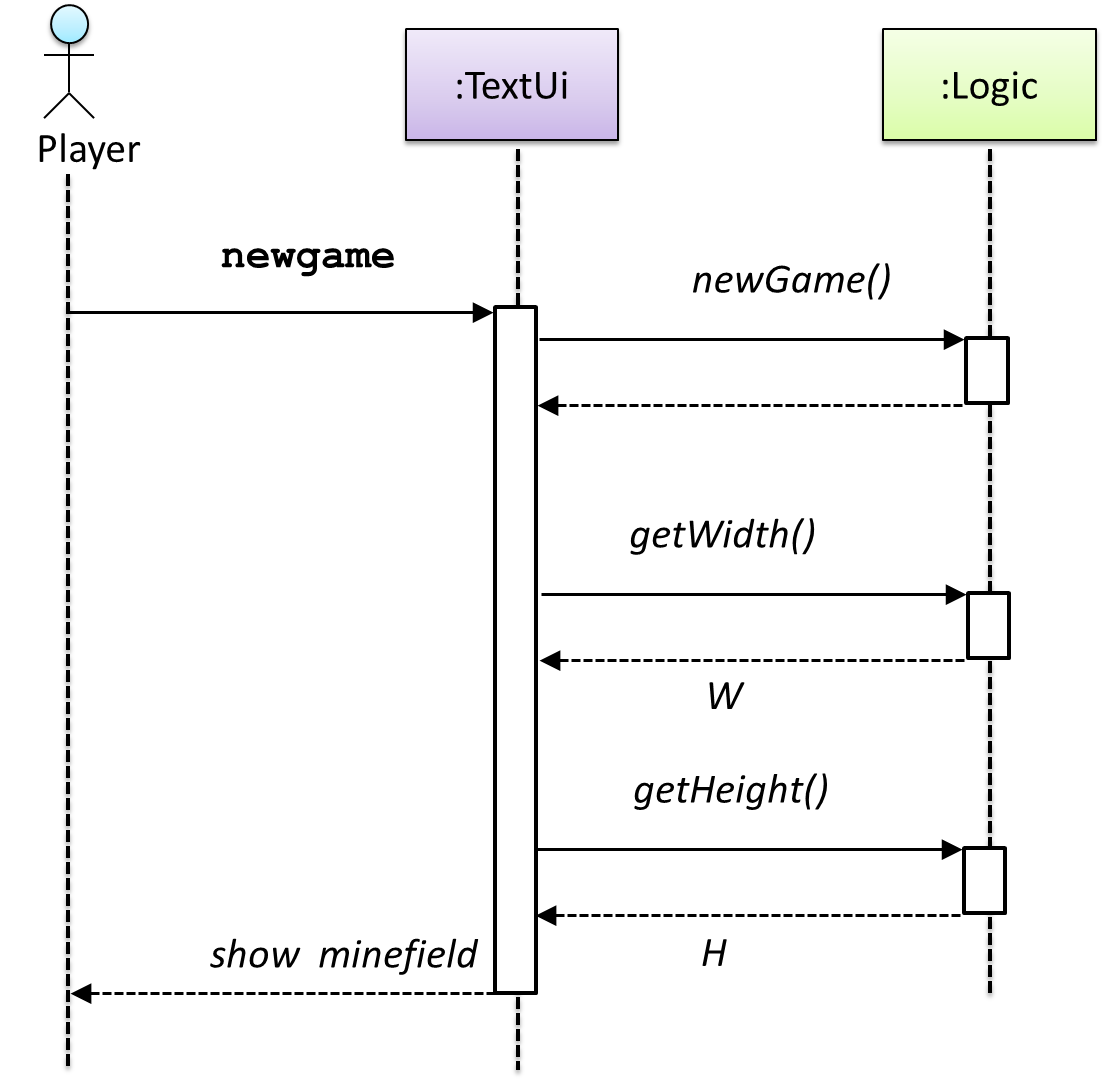`W` = Width of the minefield; `H` = Height of the minefield

The above diagram assumes that `W` and `H` are the only information `TextUi` requires to display the minefield to the `Player`. Note that there could be other ways of doing this.

The `Logic` methods you conceptualized in our modeling so far are: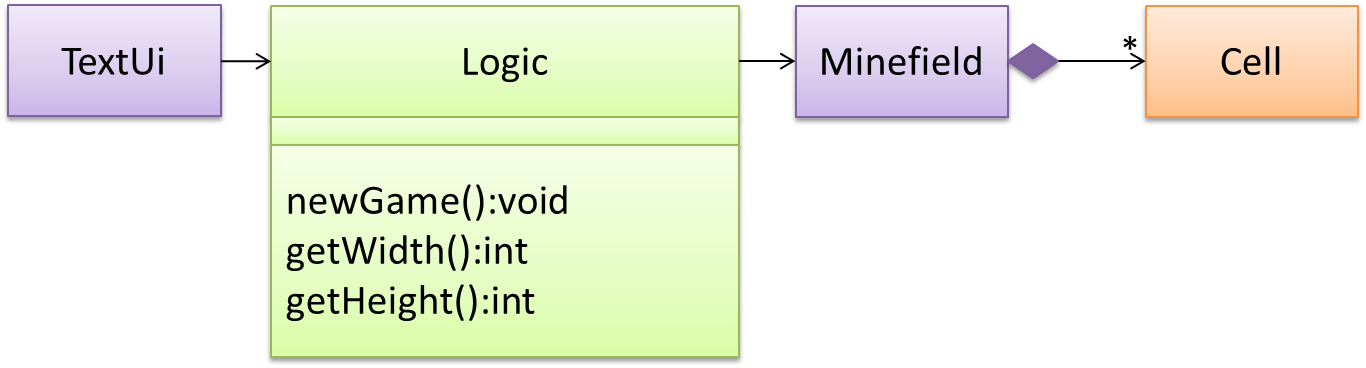Now, let us look at what other objects and interactions are needed to support the `newGame()` operation. It is likely that a new `Minefield` object is created when the `newGame()` method is called.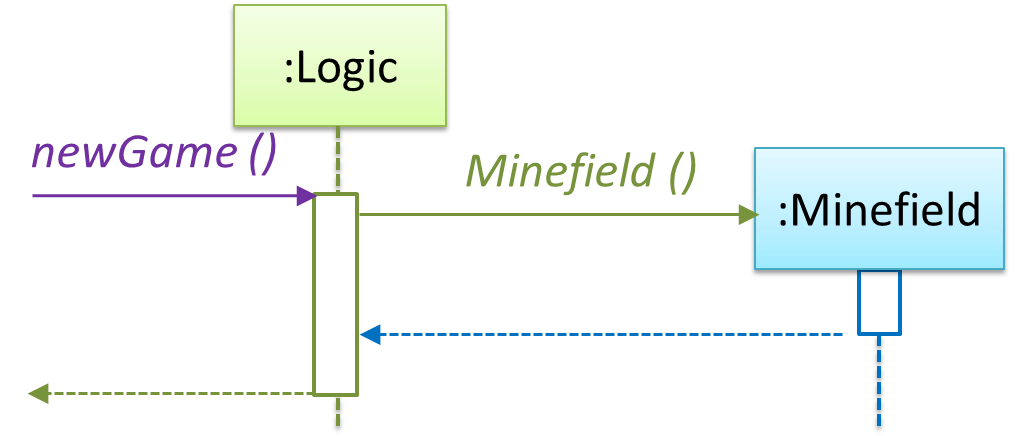Note that the behavior of the `Minefield` constructor has been abstracted away. It can be designed at a later stage.

Given below are the interactions between the player and the `TextUi` for the whole game.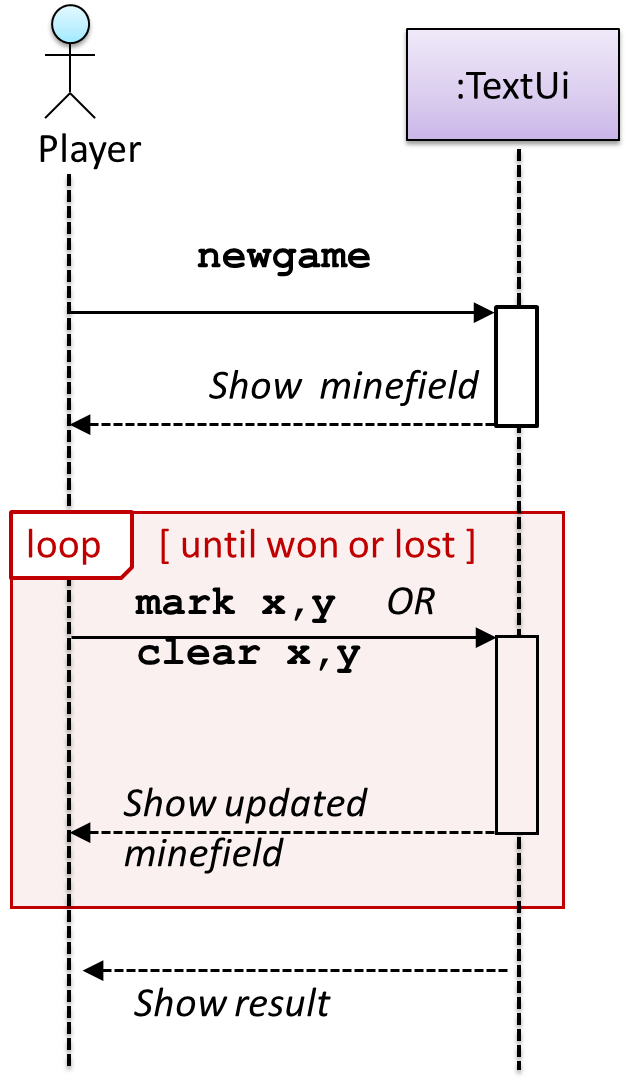Note that can be used when discovering/defining the architecture-level APIs.

Defining the architecture-level APIs for a small Tic-Tac-Toe game: